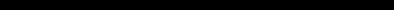# SINCLAIR ZX SPECTRUM BASIC ProgrammingCHAPTER 4
Looping

#### Summary

FOR, NEXT, TO, STEP

Suppose you want to input five numbers and add them together. One way (don't type this in unless you are feeling dutiful) is to write

10 LET total=0
20 INPUT a
30 LET total=total+a
40 INPUT a
50 LET total=total+a
60 INPUT a
70 LET total=total+a
80 INPUT a
90 LET total=total+a
100 INPUT a
110 LET total=total+a
120 PRINT total

This method is not good programming practice. It may be just about controllable for five numbers, but you can imagine how tedious a program like this to add ten numbers would be, and to add a hundred would be just impossible.

Much better is to set up a variable to count up to 5 and then stop the program, like this (which you should type in):

10 LET total=0
20 LET count=1
30 INPUT a
40 REM count=number of times that a has been input so far
50 LET total=total+a
60 LET count=count+1
70 IF count<=5 THEN GO TO 30
80 PRINT TOTAL

Notice how easy it would be to change line 70 so that this program adds ten numbers, or even a hundred.

This sort of counting is so useful that there are two special commands to make it easier: the FOR command and the NEXT command. They are always used together. Using these, the program you have just typed in does exactly the same as

10 LET total=0
20 FOR c=1 TO 5
30 INPUT a
40 REM c=number of times that a has been input so far
50 LET total=total+a
60 NEXT c
80 PRINT total

(To get this program from the previous one, you just have to edit lines 20, 40, 60, and 70. TO is SYMBOL SHIFT with F.)

Note that we have changed count to c. The counting variable - or control variable of a FOR - NEXT loop must have a single letter for its name.

The effect of this program is that c runs through the values 1 (the initial value), 2, 3, 4 and 5 (the limit), and for each one, lines 30, 40 and 50 are executed. Then, when c has finished its five values, line 80 is executed.

An extra subtlety to this is that the control variable does not have to go up by 1 each time: you can change this 1 to anything you like by using a STEP part in the FOR command. The most general form for a FOR command is

FOR control variable = initial value TO limit STEP step

where the control variable is a single letter, and the initial value, limit and step are all things that the computer can calculate as numbers - like the actual numbers themselves, or sums, or the names of numeric variables. So, if you replace line 20 in the program by

20 FOR c=1 TO 5 STEP 3/2

then c will run through the values 1, 2.5 and 4. Notice that you don't have to restrict yourself to whole numbers, and also that the control value does not have to hit the limit exactly - it carries on looping as long as it is less than or equal to the limit.

Try this program, to print out the numbers from 1 to 10 in reverse order.

10 FOR n=10 TO 1 STEP -1
20 PRINT n
30 NEXT n

We said before that the program carries on looping as long as the control variable is less than or equal to the limit. If you work out what this would mean in this case. you will see that it gives nonsense. The normal rule has to be modified: when the step is negative, the program carries on looping as long as the control variable is greater than or equal to the limit.

You must be careful if you are running two FOR - NEXT loops together, one inside the other. Try this program, which prints out the numbers for a complete set of six spot dominoes.

 10 FOR m=0 TO 6 } 20 FOR n=0 TO m } } 30 PRINT m;":";n;" "; } n-loop } 40 NEXT n } } m-loop 50 PRINT } 60 NEXT m }

You can see that the n-loop is entirely inside the m-loop - they are properly nested. What must be avoided is having two FOR - NEXT loops that overlap without either being entirely inside the other, like this:

 5 REM this program is wrong 10 FOR m=0 TO 6 } 20 FOR n=0 TO m } } 30 PRINT m;":";n;" "; } m-loop } 40 NEXT m } } n-loop 50 PRINT } 60 NEXT n }

Two FOR - NEXT loops must either be one inside the other, or be completely separate.

Another thing to avoid is jumping into the middle of a FOR - NEXT loop from the outside. The control variable is only set up properly when its FOR statement is executed, and if you miss this out the NEXT statement will confuse the computer. You will probably get an error report saying NEXT without FOR or variable not found.

There is nothing whatever to stop you using FOR and NEXT in a direct command. For example, try:

FOR m=0 TO 10: PRINT m: NEXT m

You can sometimes use this as a (somewhat artificial) way of getting round the restriction that you cannot GO TO anywhere inside a command - because a command has no line number. For instance,

FOR m=0 TO 1 STEP 0: INPUT a: PRINT a: NEXT m

The step of zero here makes the command repeat itself forever.

This sort of thing is not really recommended, because if an error crops up then you have lost the command and will have to type it in again - and CONTINUE will not work.

#### Exercises